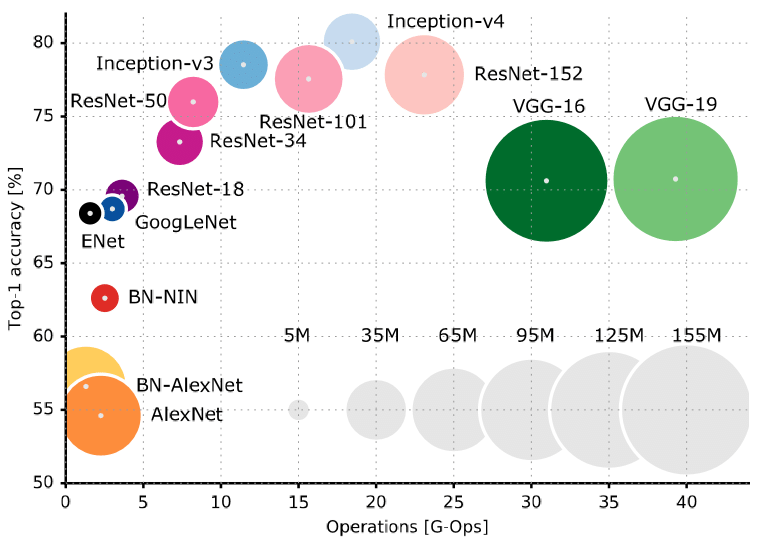# 简介

CNN -> Convolutional Neural Network

# 结构组成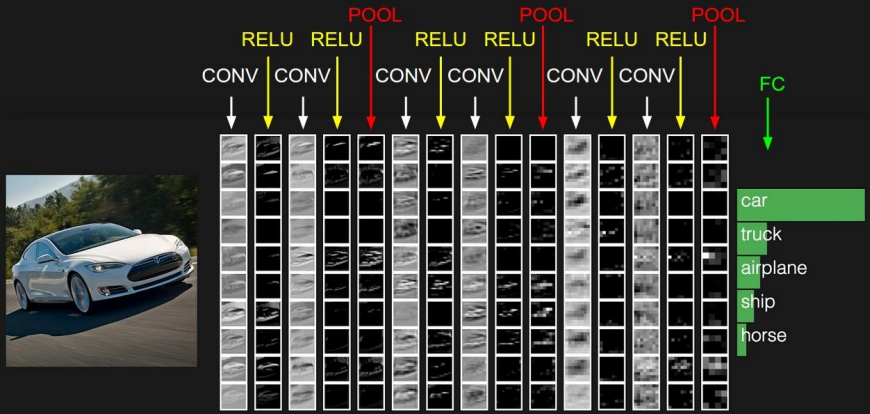## 卷积层

### 卷积计算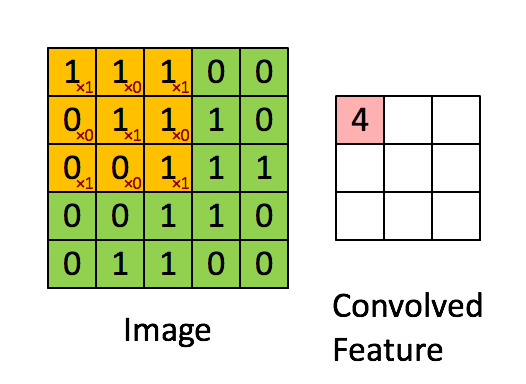NOTE: 深度学习中的卷积与信号处理中的卷积略有不同，深度学习中的卷积略去了翻转的步骤（因为起初卷积核是随机生成的，没有方向）

• 输入矩阵大小 n
• 卷积核大小 f
• 步长 (s)tride

## 池化层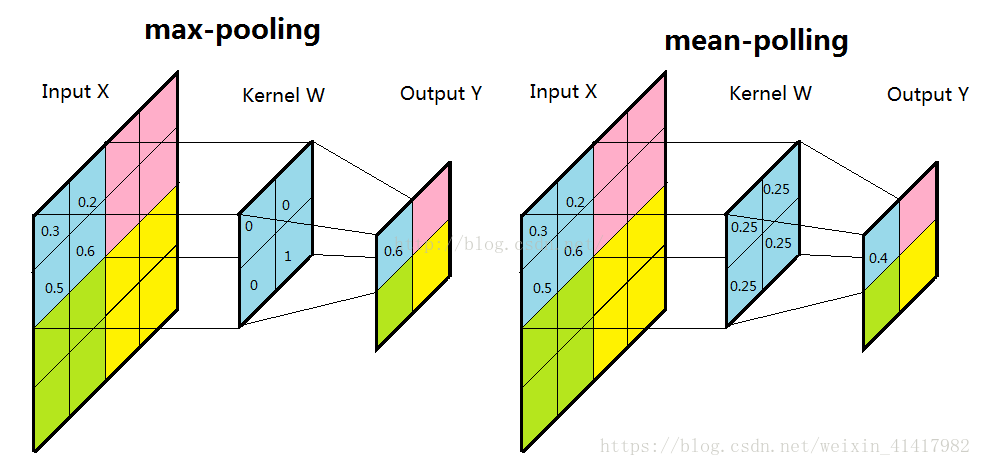## dropout层

dropout是2014年 Hinton 提出防止过拟合而采用的trick，增强了模型的泛化能力 Dropout（随机失活）是指在深度学习网络的训练过程中，按照一定的概率将一部分神经网络单元暂时从网络中丢弃，相当于从原始的网络中找到一个更瘦的网络，说的通俗一点，就是随机将一部分网络的传播掐断，听起来好像不靠谱，但是通过实际测试效果非常好。

# 经典模型

## LeNet-5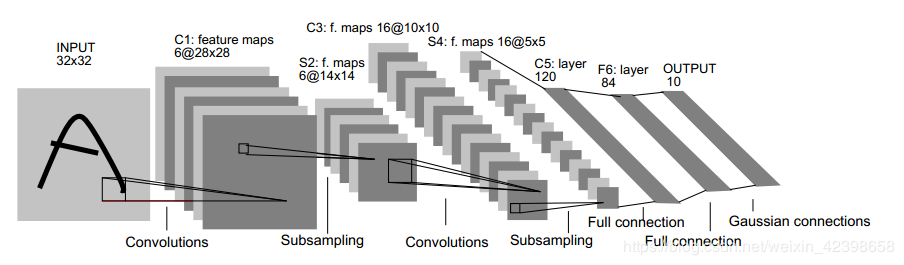• 用卷积提取空间特征；
• 由空间平均得到子样本；
• 用 tanh 或 sigmoid 得到非线性；
• 用 multi-layer neural network（MLP）作为最终分类器；
• 层层之间用稀疏的连接矩阵，以避免大的计算成本。

## AlexNet

• 更深的网络结构
• 用ReLu替换之前的Sigmoid作为激活函数
• 使用Dropout抑制过拟合
• 使用数据增强Data Augmentation抑制过拟合
• 使用层叠的卷积层，即卷积层+卷积层+池化层来提取图像的特征
• 重叠最大池，避免平均池的平均效果；
• 使用 GPU NVIDIA GTX 580 可以减少训练时间，这比用CPU处理快了 10 倍，所以可以被用于更大的数据集和图像上。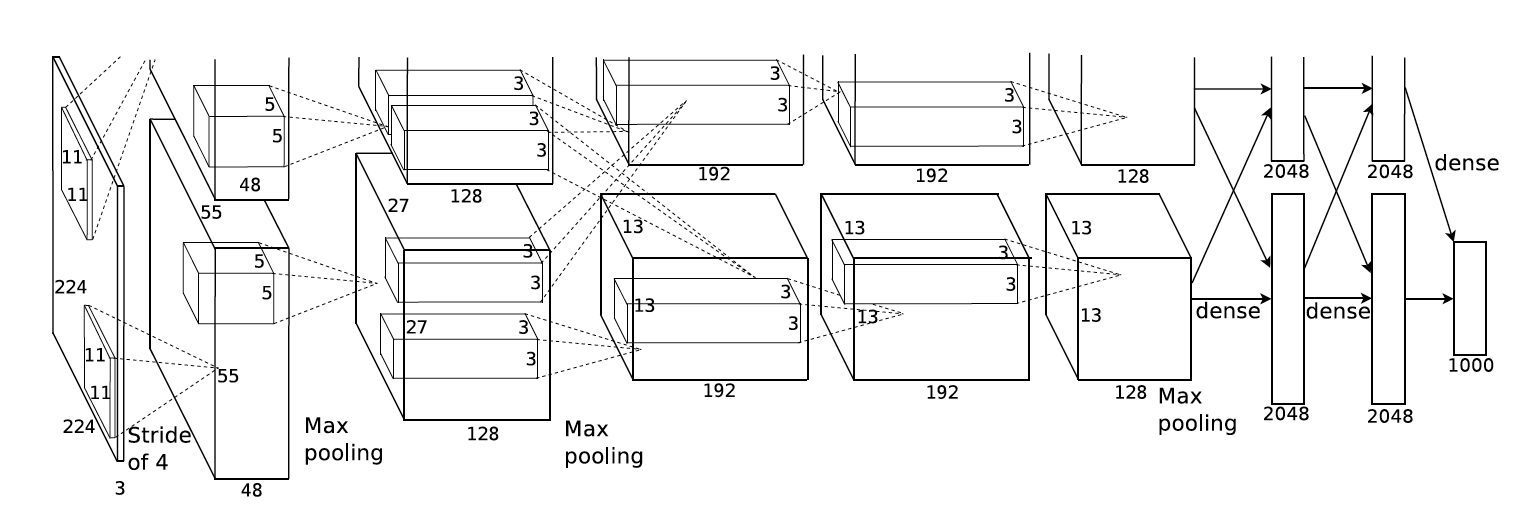AlexNet有一个特殊的计算层：LRN(Local Response Normalized层)，这一层用于对当前层的输出结果做平滑处理

Alexnet的每一阶段（含一次卷积主要计算的算作一层）可以分为8层：

1. 卷积层1 `Conv-Relu-Pooling-LRN`

1. 卷积层2 `Conv-Relu-Pooling-LRN`

1. 卷积层3 `Conv-Relu`

1. 卷积层4 `Conv-Relu`

1. 卷积层5 `Conv-Relu-Pooling`

1. 全连接层1 `fc-Relu-Dropout`

Dropout层：在训练中以50%的概率使得隐藏层的某些neuron的输出为0，这样就都丢掉了一半节点的输出，BP的时候也不会更新这些节点，防止过拟合

1. 全连接层2 `fc-Relu-Dropout`

2. 全连接层3 `fc-softmax`

Pytorch的`torchvision`包中包含AlexNet的官方实现

``````import torcvision
model = torchvision.models.alexnet(pretrained=False)
print(model)
'''
AlexNet(
(features): Sequential(
(0): Conv2d(3, 64, kernel_size=(11, 11), stride=(4, 4), padding=(2, 2))
(1): ReLU(inplace=True)
(2): MaxPool2d(kernel_size=3, stride=2, padding=0, dilation=1, ceil_mode=False)
(3): Conv2d(64, 192, kernel_size=(5, 5), stride=(1, 1), padding=(2, 2))
(4): ReLU(inplace=True)
(5): MaxPool2d(kernel_size=3, stride=2, padding=0, dilation=1, ceil_mode=False)
(6): Conv2d(192, 384, kernel_size=(3, 3), stride=(1, 1), padding=(1, 1))
(7): ReLU(inplace=True)
(8): Conv2d(384, 256, kernel_size=(3, 3), stride=(1, 1), padding=(1, 1))
(9): ReLU(inplace=True)
(10): Conv2d(256, 256, kernel_size=(3, 3), stride=(1, 1), padding=(1, 1))
(11): ReLU(inplace=True)
(12): MaxPool2d(kernel_size=3, stride=2, padding=0, dilation=1, ceil_mode=False)
)
(classifier): Sequential(
(0): Dropout(p=0.5, inplace=False)
(1): Linear(in_features=9216, out_features=4096, bias=True)
(2): ReLU(inplace=True)
(3): Dropout(p=0.5, inplace=False)
(4): Linear(in_features=4096, out_features=4096, bias=True)
(5): ReLU(inplace=True)
(6): Linear(in_features=4096, out_features=1000, bias=True)
)
)
'''
``````

## VGG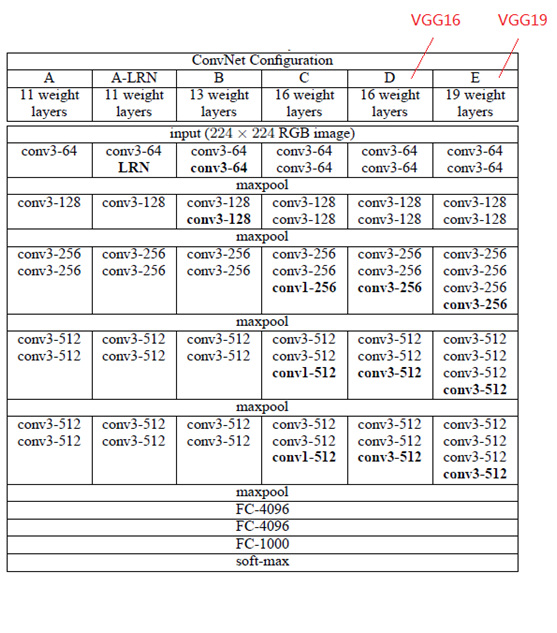• 每个卷积层中使用更小的 3×3 filters，并将它们组合成卷积序列
• 多个3×3卷积序列可以模拟更大的接收场的效果
• 每次的图像像素缩小一倍，卷积核的数量增加一倍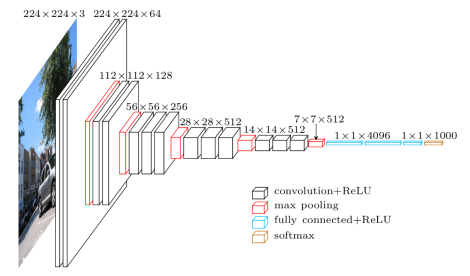VGG由5层卷积层、3层全连接层、softmax输出层构成，层与层之间为max-pooling，所有隐层的激活函数均为ReLu

1. 小卷积核

VGG采用多个小卷积核(3x3)的卷积层代替一个卷积核较大的卷积层。这样一方面可以减少卷积层的参数，另一方面相当于进行了更多的非线性映射，可以增加网络的拟合/表达能力

VGG的作者认为，当input为8x8，经过三层Conv 3x3后，output为2x2，这等同于1层Conv 7x7的结果；当input为8x8，经过2层Conv 3x3后，output为2x2，等同于1层Conv 5x5的结果。这样可以增加非线性映射，也能很好地减少参数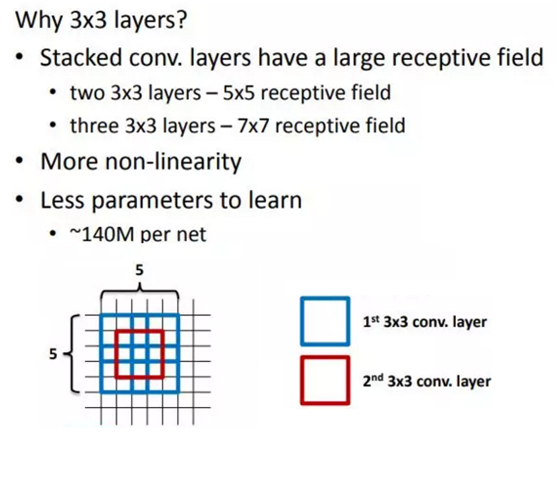1. 小池化核

1. 通道数多

VGG网络第一层的通道数为64，后面每层都进行了翻倍，最多到512个通道，通道数的增加，使得更多的信息可以被提取出来

1. 层数更深、特征图更宽

``````import torchvision
model = torchvision.models.vgg16(pretrained=False)
print(model)
'''
VGG(
(features): Sequential(
(0): Conv2d(3, 64, kernel_size=(3, 3), stride=(1, 1), padding=(1, 1))
(1): ReLU(inplace)
(2): Conv2d(64, 64, kernel_size=(3, 3), stride=(1, 1), padding=(1, 1))
(3): ReLU(inplace)
(4): MaxPool2d(kernel_size=2, stride=2, padding=0, dilation=1, ceil_mode=False)
(5): Conv2d(64, 128, kernel_size=(3, 3), stride=(1, 1), padding=(1, 1))
(6): ReLU(inplace)
(7): Conv2d(128, 128, kernel_size=(3, 3), stride=(1, 1), padding=(1, 1))
(8): ReLU(inplace)
(9): MaxPool2d(kernel_size=2, stride=2, padding=0, dilation=1, ceil_mode=False)
(10): Conv2d(128, 256, kernel_size=(3, 3), stride=(1, 1), padding=(1, 1))
(11): ReLU(inplace)
(12): Conv2d(256, 256, kernel_size=(3, 3), stride=(1, 1), padding=(1, 1))
(13): ReLU(inplace)
(14): Conv2d(256, 256, kernel_size=(3, 3), stride=(1, 1), padding=(1, 1))
(15): ReLU(inplace)
(16): MaxPool2d(kernel_size=2, stride=2, padding=0, dilation=1, ceil_mode=False)
(17): Conv2d(256, 512, kernel_size=(3, 3), stride=(1, 1), padding=(1, 1))
(18): ReLU(inplace)
(19): Conv2d(512, 512, kernel_size=(3, 3), stride=(1, 1), padding=(1, 1))
(20): ReLU(inplace)
(21): Conv2d(512, 512, kernel_size=(3, 3), stride=(1, 1), padding=(1, 1))
(22): ReLU(inplace)
(23): MaxPool2d(kernel_size=2, stride=2, padding=0, dilation=1, ceil_mode=False)
(24): Conv2d(512, 512, kernel_size=(3, 3), stride=(1, 1), padding=(1, 1))
(25): ReLU(inplace)
(26): Conv2d(512, 512, kernel_size=(3, 3), stride=(1, 1), padding=(1, 1))
(27): ReLU(inplace)
(28): Conv2d(512, 512, kernel_size=(3, 3), stride=(1, 1), padding=(1, 1))
(29): ReLU(inplace)
(30): MaxPool2d(kernel_size=2, stride=2, padding=0, dilation=1, ceil_mode=False)
)
(classifier): Sequential(
(0): Linear(in_features=25088, out_features=4096, bias=True)
(1): ReLU(inplace)
(2): Dropout(p=0.5)
(3): Linear(in_features=4096, out_features=4096, bias=True)
(4): ReLU(inplace)
(5): Dropout(p=0.5)
(6): Linear(in_features=4096, out_features=1000, bias=True)
)
)
'''
``````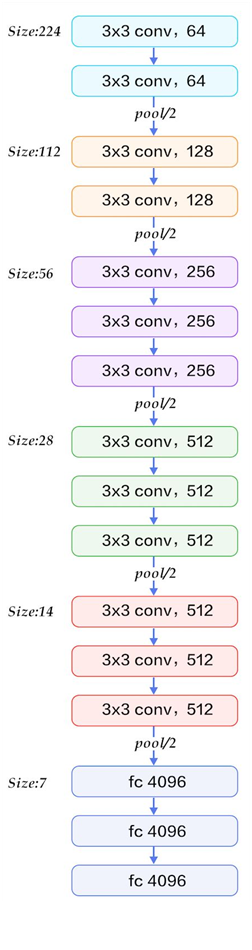### Inception V1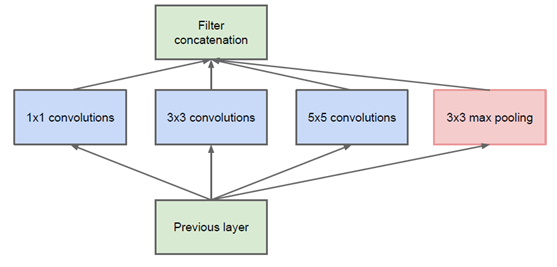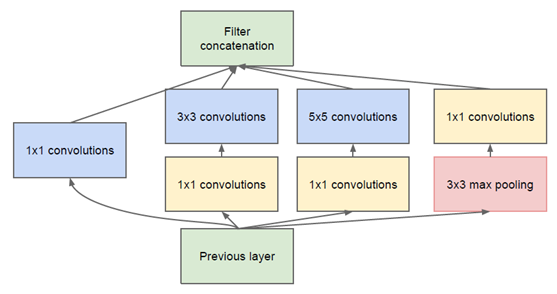1x1卷积的主要目的是为了减少维度，还用于修正线性激活（ReLU）。

• 使用1x1的卷积块(NIN)来减少特征数量，这通常被称为“瓶颈”，可以减少深层神经网络的计算负担
• 每个池化层之前，增加feature maps， 增加每一层的宽度来增多特征的组合性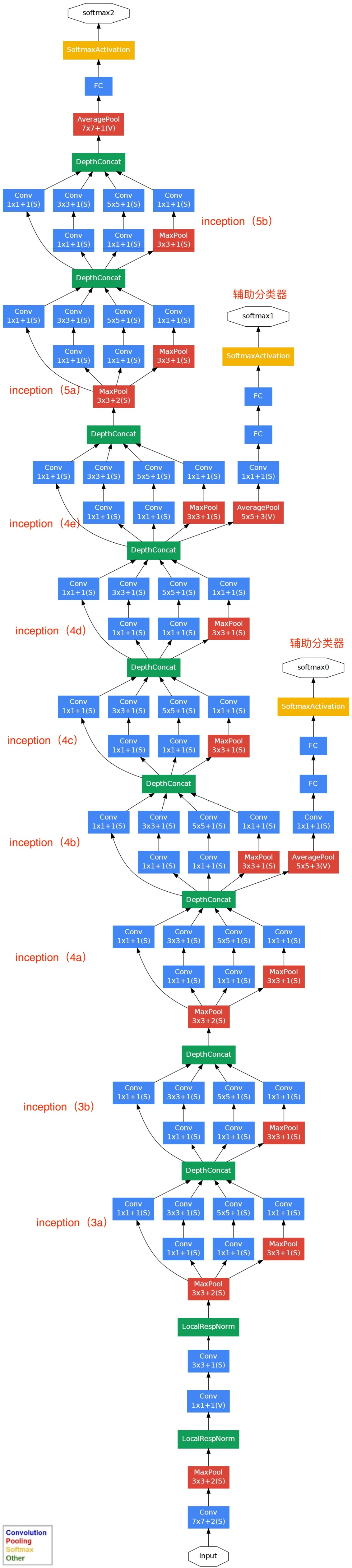Inception架构的主要思想是找出如何让已有的稠密组件接近与覆盖卷积视觉网络中的最佳局部稀疏结构。

``````# inception_v3需要scipy
import torchvision
model = torchvision.models.inception_v3(pretrained=False) #我们不下载预训练权重
print(model)
'''

'''
``````

## ResNet

### 退化问题

#### 残差网络的解决办法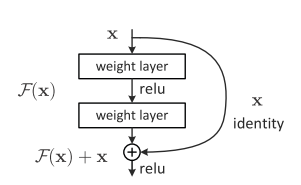### 网络结构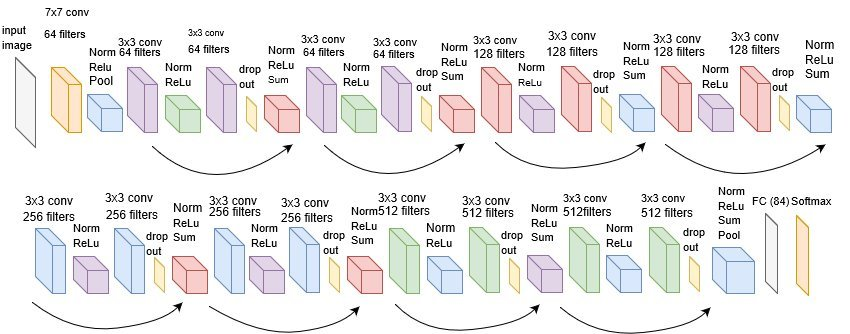``````import torchvision
model = torchvision.models.resnet18(pretrained=False) #我们不下载预训练权重
print(model)
``````

# 选择模型